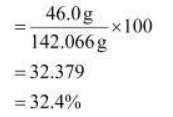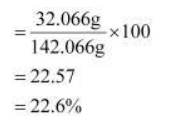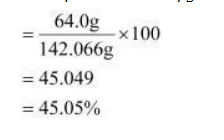# Calculate the mass percent of different elementsQuestion.

Calculate the mass percent of different elements present in sodium sulphate $\left(\mathrm{Na}_{2} \mathrm{SO}_{4}\right)$.

Solution:

The molecular formula of sodium sulphate is $\mathrm{Na}_{2} \mathrm{SO}_{4}$.

Molar mass of $\mathrm{Na}_{2} \mathrm{SO}_{4}=[(2 \times 23.0)+(32.066)+4(16.00)]$

$=142.066 \mathrm{~g}$

Mass percent of an element $=\frac{\text { Mass of that element in the compound }}{\text { Molar mass of the compound }} \times 100$

Mass percent of sodiumMass percent of sulphurMass percent of oxygen